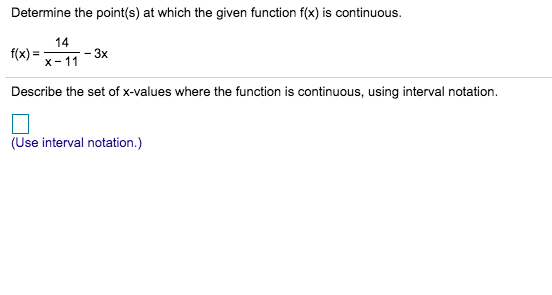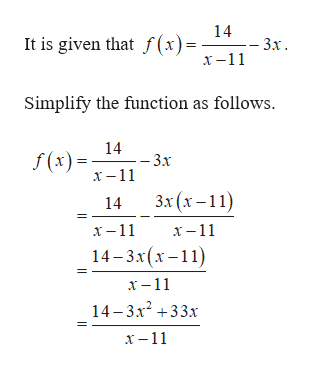Determine the point(s) at which the given function f(x) is continuous.14-3xX 11f(x)Describe the set of x-values where the function is continuous, using interval notation.(Use interval notation.)

Question

Can i get help with this problem step by step?help_outlineImage TranscriptioncloseDetermine the point(s) at which the given function f(x) is continuous. 14 -3x X 11 f(x) Describe the set of x-values where the function is continuous, using interval notation. (Use interval notation.) fullscreen
Step 1

Consider the given...help_outlineImage Transcriptionclose14 - 3х. It is given that f(x)= -11 Simplify the function as follows 14 -3x f(x)11 Зx (x-11) 14 х -11 х -11 14-3x(x-11 х -11 14-3x233x х —11 fullscreen

Want to see the full answer?

See Solution

Want to see this answer and more?

Our solutions are written by experts, many with advanced degrees, and available 24/7

See Solution
Tagged in

Other